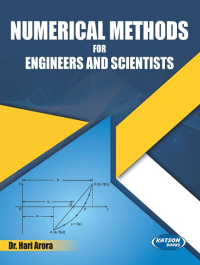•## Numerical Methods for Engineers and Scientists

In Stock
ISBN 978-93-5014-729-0 Dr. Hari Arora S.K. Kataria & Sons 1st 2021 2021 525 Print In Stock
Rs 475.00Rs 428.00

#### CONTENTS

• Errors and Approximations in Numerical Computations • Solution of Algebraic and Transcendental Equations • Solution of Simultaneous Algebraic Equations • Matrix Inversion and Eigen Value Problem • Curve Fitting and Method of Least Squares • Calculus of Finite Differences • Interpolation with Equal and Unequal Intervals • Numerical Differentiation • Numerical Integration • Numerical Solution of Ordinary Differential Equations • Numerical Solution of Partial Differential Equations • Index.

• Author : Dr. Hari Arora
• Publisher : S.K. Kataria & Sons
• Edition : 1st 2021
• Publishing Year : 2021
• Total Pages : 525